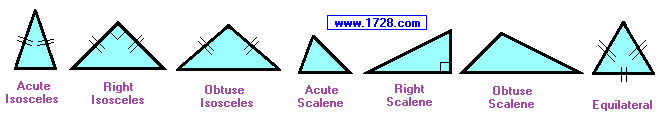Acute, Right, Obtuse Triangle Tester Note: If you are given 3 angles and they sum to 180° they will always form a triangle. Input Triangle Sides       A= B= C=                     Sum of the squares of 2 smallest sides =               Longest Side Squared =The above graphic shows all 7 possible triangles that can exist.
 CALCULATOR   INSTRUCTIONS Input 3 triangle side lengths (A, B and C), then click "ENTER". This calculator will determine whether those 3 sides will form an equilateral, isoceles, acute, right or obtuse triangle or no triangle at all. Without Using The Calculator When given 3 triangle sides, to determine if the triangle is acute, right or obtuse: 1) Square all 3 sides. 2) Sum the squares of the 2 shortest sides. 3) Compare this sum to the square of the 3rd side. if sum > (3rd side)2   Acute Triangle if sum = (3rd side)2   Right Triangle if sum < (3rd side)2   Obtuse Triangle
 Here are three examples: 5, 6 and 7 Each side squared = 25, 36 and 49 sum of the squares of the short sides = 25 + 36 = 61 61 > 49 Acute Triangle 3, 4 and 5 Squaring each side = 9, 16 and 25 sum of the squares of the short sides = 9 + 16 = 25 25 = 25 Right Triangle 3, 7 and 9 Squaring each side = 9, 49 and 81 sum of the squares of the short sides = 9 + 49 = 58 58 < 81 Obtuse Triangle For determining if the 3 sides can even form a triangle, the triangle inequality theorem states that the longest side must be shorter than the sum of the other 2 sides.